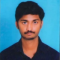## Solve the following equation and also verify your solution:$\frac{2}{3}(x-5)-\frac{1}{4}(x-2)=\frac{9}{2}$Akhileshwar Nani

Updated on 13-Apr-2023 23:02:58

93 Views

Given:The given equation is $\frac{2}{3}(x-5)-\frac{1}{4}(x-2)=\frac{9}{2}$.To do:We have to solve the given equation and verify the solution.Solution:To verify the solution we have to find the value of the variable and substitute it in the equation. Find the value of LHS and the value of RHS and check whether both are equal.The given ... Read More

## Solve each of the following equations and also verify your solution:(i) $\frac{(2x-1)}{3}-\frac{(6x-2)}{5}=\frac{1}{3}$(ii) $13(y-4)-3(y-9)-5(y+4)=0$Akhileshwar Nani

Updated on 13-Apr-2023 23:02:13

129 Views

Given:The given equations are:(i) $\frac{(2x-1)}{3}-\frac{(6x-2)}{5}=\frac{1}{3}$(ii) $13(y-4)-3(y-9)-5(y+4)=0$To do:We have to solve the given equations and verify the solutions.Solution:To verify the solutions we have to find the values of the variables and substitute them in the equation. Find the value of LHS and the value of RHS and check whether both are ... Read More

## Solve each of the following equations and also verify your solution:(i) $\frac{x}{2}-\frac{4}{5}+\frac{x}{5}+\frac{3x}{10}=\frac{1}{5}$(ii) $\frac{7}{x}+35=\frac{1}{10}$Akhileshwar Nani

Updated on 13-Apr-2023 23:01:43

67 Views

Given:The given equations are:(i) $\frac{x}{2}-\frac{4}{5}+\frac{x}{5}+\frac{3x}{10}=\frac{1}{5}$(ii) $\frac{7}{x}+35=\frac{1}{10}$To do:We have to solve the given equations and verify the solutions.Solution:To verify the solutions we have to find the values of the variables and substitute them in the equation. Find the value of LHS and the value of RHS and check whether both are ... Read More

## Solve each of the following equations and also verify your solution:(i) $\frac{2x}{3}-\frac{3x}{8}=\frac{7}{12}$(ii) $(x+2)(x+3)+(x-3)(x-2)-2x(x+1)=0$Akhileshwar Nani

Updated on 13-Apr-2023 23:00:44

74 Views

Given:The given equations are:(i) $\frac{2x}{3}-\frac{3x}{8}=\frac{7}{12}$(ii) $(x+2)(x+3)+(x-3)(x-2)-2x(x+1)=0$To do:We have to solve the given equations and verify the solutions.Solution:To verify the solutions we have to find the values of the variables and substitute them in the equation. Find the value of LHS and the value of RHS and check whether both are ... Read More

## Solve each of the following equations and also verify your solution:(i) $\frac{x}{2}+\frac{x}{3}+\frac{x}{4}=13$(ii) $\frac{x}{2}+\frac{x}{8}=\frac{1}{8}$Akhileshwar Nani

Updated on 13-Apr-2023 23:00:10

51 Views

Given:The given equations are:(i) $\frac{x}{2}+\frac{x}{3}+\frac{x}{4}=13$(ii) $\frac{x}{2}+\frac{x}{8}=\frac{1}{8}$To do:We have to solve the given equations and verify the solutions.Solution:To verify the solutions we have to find the values of the variables and substitute them in the equation. Find the value of LHS and the value of RHS and check whether both are ... Read More

## Solve each of the following equations and also verify your solution:(i) $9\frac{1}{4}=y-1\frac{1}{3}$(ii) $\frac{5x}{3}+\frac{2}{5}=1$Akhileshwar Nani

Updated on 13-Apr-2023 22:59:30

81 Views

Given:The given equations are:(i) $9\frac{1}{4}=y-1\frac{1}{3}$(ii) $\frac{5x}{3}+\frac{2}{5}=1$To do:We have to solve the given equations and verify the solutions.Solution:To verify the solutions we have to find the values of the variables and substitute them in the equation. Find the value of LHS and the value of RHS and check whether both are ... Read More

## Divide:(i) $acx^2+(bc+ad)x+bd$ by $ax+b$(ii) $(a^2+2ab+b^2)-(a^2+2ac+c^2)$ by $2a+b+c$Akhileshwar Nani

Updated on 13-Apr-2023 22:58:49

38 Views

Given:The given expressions are:(i) $acx^2+(bc+ad)x+bd$ by $ax+b$(ii) $(a^2+2ab+b^2)-(a^2+2ac+c^2)$ by $2a+b+c$To do:We have to divide the given expressions.Solution:We have to divide the given polynomials by simplifying them using algebraic formulas.Polynomials: Polynomials are expressions in which each term is a constant multiplied by a variable raised to a whole number power.Therefore, (i) The given ... Read More

## Divide:(i) $ax^2-ay^2$ by $ax+ay$(ii) $x^4-y^4$ by $x^2-y^2$Akhileshwar Nani

Updated on 13-Apr-2023 22:58:01

62 Views

Given:The given expressions are:(i) $ax^2-ay^2$ by $ax+ay$(ii) $x^4-y^4$ by $x^2-y^2$To do:We have to divide the given expressions.Solution:We have to divide the given polynomials by simplifying them using algebraic formulas.Polynomials: Polynomials are expressions in which each term is a constant multiplied by a variable raised to a whole number power.Therefore, (i) The given ... Read More

## Divide:(i) $5x^3-15x^2+25x$ by $5x$(ii) $4z^3+6z^2-z$ by $\frac{-1}{2}z$(iii) $9x^2y-6xy+12xy^2$ by $\frac{-3}{2}xy$Akhileshwar Nani

Updated on 13-Apr-2023 22:56:43

131 Views

Given:The given expressions are:(i) $5x^3-15x^2+25x$ by $5x$(ii) $4z^3+6z^2-z$ by $\frac{-1}{2}z$(iii) $9x^2y-6xy+12xy^2$ by $\frac{-3}{2}xy$To do:We have to divide the given expressions.Solution:We have to divide the given polynomials by monomials using the formula $x^a \div x^b=a^{a-b}$Polynomials: Polynomials are expressions in which each term is a constant multiplied by a variable raised to a ... Read More

## Divide:(i) $-x^6+2x^4+4x^3+2x^2$ by $\sqrt2x^2$(ii) $-4a^3+4a^2+a$ by $2a$(iii) $\sqrt3a^4+2\sqrt3a^3+3a^2-6a$ by $3a$Akhileshwar Nani

Updated on 13-Apr-2023 22:55:41

41 Views

Given:The given expressions are:(i) $-x^6+2x^4+4x^3+2x^2$ by $\sqrt2x^2$(ii) $-4a^3+4a^2+a$ by $2a$(iii) $\sqrt3a^4+2\sqrt3a^3+3a^2-6a$ by $3a$To do:We have to divide the given expressions.Solution:We have to divide the given polynomials by monomials using the formula $x^a \div x^b=a^{a-b}$Polynomials: Polynomials are expressions in which each term is a constant multiplied by a variable raised to a ... Read More